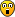Screenshots, descriptions and links to applications compiled with LB Booster, to illustrate how it is being used
JackKelly
Posts: 52
Joined: Fri Apr 06, 2018 2:38 am
Location: Rome NY, USAHere's a fun program to test the IQ of your programs. It reports a raw score primarily based on the number of logical expressions in the .bas file. Most of the complexity is ensuring that conditional keywords are not accidently picked up in remarks or strings. Lately, at the urging of Richard, I have been trying hard to eliminate GOTOs and labels from my programs. I couldn't figure out a graceful way, though, to eliminate them from this program. I would really like to have the Liberty BASIC language support multiple NEXTs in FOR loops, LOOPs in DO loops, and WENDs in WHILE loops, in addition to EXITs of course.

Tentatively I think in this program a raw score of 20 might equate to an average IQ of 100. The highest possible IQ should be 200. The program is LB4.5 and JB compatible, so everyone can test their best programs. My highest scoring program was 802, which might equate to an IQ of around 175. I would be grateful if anyone can suggest an algebraic equation to calculate the IQ from the raw score. I'm sure such an equation would have something to do with logarithms and exponents.Regards,
Jack

Code: Select all

``````mainwin 120 30
titlebar "Program IQ Test"
filedialog "Open .BAS File for IQ Testing", "*.bas", SelectedFile\$
if SelectedFile\$="" then goto [Stop]
titlebar SelectedFile\$
open SelectedFile\$ for input as #1
do while not(EOF(#1))
line input #1, FileRec\$
WordNum=0
W\$=""
String=0
do
PW\$=W\$
WordNum=WordNum+1
W\$=lower\$(word\$(FileRec\$, WordNum))
if W\$="" then print "|X": exit do 'end of a line
if left\$(W\$, 1)="'" then print "|'": exit do 'start of a remark
if left\$(W\$, 1)=chr\$(34) then
String=1 'start of a string
print "|\$>"; W\$;
if instr(W\$, chr\$(34), 2) then
String=0 'end of the one word string
print "<\$";
end if
goto [loop]
end if
if String=1 then
if instr(W\$, chr\$(34), 1) then
String=0 'end of the multiword string
print "|"; W\$; "<\$";
else
print "|"; W\$;
end if
goto [loop]
end if
print "|"; W\$;
if right\$(W\$, 1)="]" then W\$="[]"
select case W\$
case "[]"
print "<B";: IQ=IQ-1
case "function"
if WordNum=1 then print "<f";: IQ=IQ+2
case "for"
if WordNum=1 then print "<F";: IQ=IQ+1
case "if"
if WordNum=1 then print "<I";: IQ=IQ+1
case "case"
if WordNum=1 then print "<C";: IQ=IQ+1
case "while"
if PW\$<>"exit" then print "<W";: IQ=IQ+1
case "until"
print "<U";: IQ=IQ+1
case "or"
print "<O";: IQ=IQ+1
case "and"
print "<A";: IQ=IQ+1
case "xor"
print "<X";: IQ=IQ+2
case "call"
print "<c";: IQ=IQ+2
end select
[loop] loop while W\$<>""
loop

[Stop]
close #1
print "Raw Score = "; IQ
print "Program Complete. Click X to close."
end
``````
AttachmentsEPSON009.jpg (62.14 KiB) Viewed 4473 times

RobM
Posts: 3
Joined: Thu Apr 05, 2018 9:50 pm

### Re: Test Your Program's IQ

29,106

JackKelly
Posts: 52
Joined: Fri Apr 06, 2018 2:38 am
Location: Rome NY, USA

### Re: Test Your Program's IQ

That's a WOW, Rob. I don't think anyone will ever beat Cabinet Planer! Thanks for posting your record breaker.